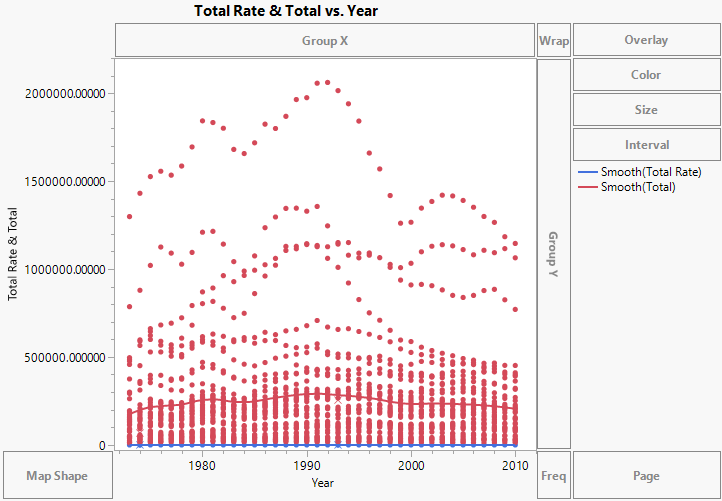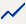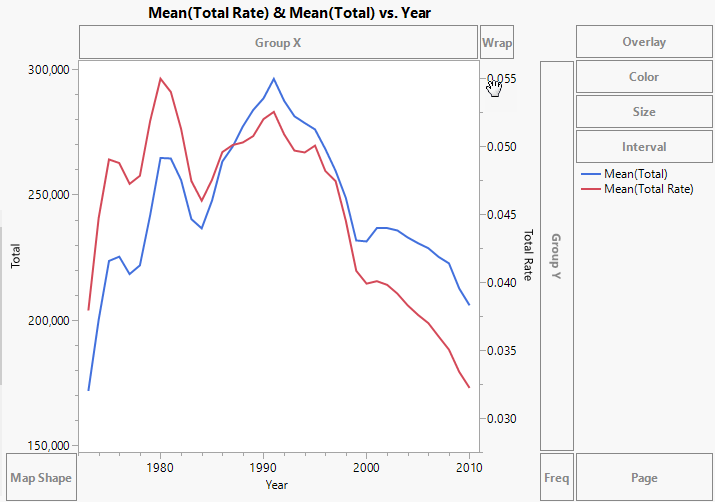Essential Graphing > Graph Builder > Work with Axes > Create a Second Y Axis
Publication date: 05/24/2021

## Create a Second Y Axis

If you have two or more Y variables on the same axis, you can reflect the scaling of a second set of variables by creating a second Y axis. This can be useful when two variables measure the same underlying quantities, but have different scales. In general, it is unwise to use a second Y axis in any other situation. See Few (2008).

To create a second Y axis:

1. Right-click one of the Y variable names in the Y zone and select Move Right.

2. Select the variable or variables that you want to move to the new axis.

The new axis is scaled according to the values of the specified variable, and the selected variable is plotted against this axis.

3. Repeat the process to plot additional variables against the new axis.

The new axis adjusts to accommodate the values of the additional variables.

#### Change the Graph for a Second Y Axis

When you click an element type, it applies only to the variables on the left Y axis. To apply an element to the variables on the right Y axis, right-click and change the second element, which corresponds to the right axis.

#### Example of Creating a Second Y Axis

The CrimeData.jmp sample data table contains data on various types of crime for the 50 US states yearly, from 1973 to 2010. The Total column sums the number of incidents, and the Total Rate column gives a population-adjusted rate. You want to show these two variables on a single chart.

1. Select Help > Sample Data Library and open CrimeData.jmp.

2. Select Graph > Graph Builder.

3. Select Total Rate and Total and drag them to the Y zone.

4. Select Year and drag it to the X zone.

Figure 3.14 Total Rate and Total MergedThe Total Rate values are all between 0 and 1, and they are barely visible. Calculate yearly means and then use a second Y axis to make these values visible.

5. In the options panel for Points, select Mean next to Summary Statistic.

6. Click the Line element.

7. Right-click the variable names in the Y zone and select Move Right > Total Rate.

Figure 3.15 Second Y Axis AddedAn axis for Total Rate is added on the right, and the axes rescale to show both sets of values. You can now see relationships between the two measures. For example, both measures began to decrease in 1991.

8. (Optional) Click Done.